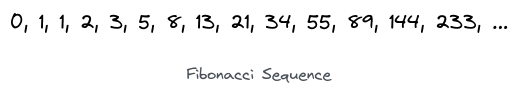# Fibonacci BetweenTough Dash, January 2023
Limits 1s, 512 MB

Given two numbers $L$ and $R$, you must print all the Fibonacci numbers between $L$ and $R$ (inclusive).Although the number 1 appears twice in the sequence, print it only once if it falls in the given range.

You can generate the Fibonacci sequence by starting with 0 and 1. And then, add the last two numbers in the sequence every time to generate the following number.

For example:

• $0 + 1 = 1$

• $1 + 1 = 2$

• $1 + 2 = 3$

• $2 + 3 = 5$

• And so on.

## Input

The input will contain two integers: $L$ and $R$ ($0 \le L < R \le 10^7$). The input will be such that at least one Fibonacci number will be within this range.

## Output

Print all Fibonacci numbers between $L$ and $R$ (inclusive).

## Sample

InputOutput
0 10

0
1
2
3
5
8


The numbers 0, 1, 2, 3, 5, 8 are part of the Fibonacci sequence and fall in the range $[0, 10]$.Math

### Submit